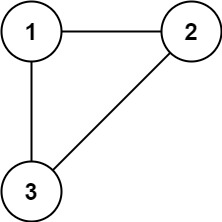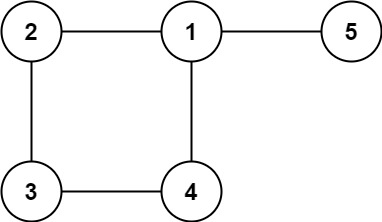# GeetCode Hub

In this problem, a tree is an undirected graph that is connected and has no cycles.

You are given a graph that started as a tree with `n` nodes labeled from `1` to `n`, with one additional edge added. The added edge has two different vertices chosen from `1` to `n`, and was not an edge that already existed. The graph is represented as an array `edges` of length `n` where `edges[i] = [ai, bi]` indicates that there is an edge between nodes `ai` and `bi` in the graph.

Return an edge that can be removed so that the resulting graph is a tree of `n` nodes. If there are multiple answers, return the answer that occurs last in the input.

Example 1:```Input: edges = [[1,2],[1,3],[2,3]]
Output: [2,3]
```

Example 2:```Input: edges = [[1,2],[2,3],[3,4],[1,4],[1,5]]
Output: [1,4]
```

Constraints:

• `n == edges.length`
• `3 <= n <= 1000`
• `edges[i].length == 2`
• `1 <= ai < bi <= edges.length`
• `ai != bi`
• There are no repeated edges.
• The given graph is connected.

class Solution { public int[] findRedundantConnection(int[][] edges) { } }